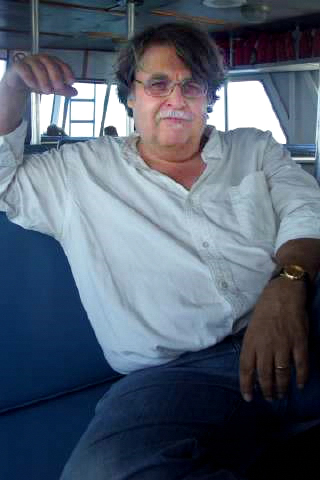#Eric Bach

Professor

Computer Sciences Department
University of Wisconsin
1210 W. Dayton St.
Madison, WI 53706-1685

telephone: (608) 262-1204
fax: (608) 262-9777
email: bach at cs dot wisc dot edu
Ph.D., University of California, Berkeley, 1984
Interests: Theoretical computer science, computational number theory, algebraic algorithms, complexity theory, cryptography, six-string automata

## Research Summary

I am interested in how one uses computers to efficiently solve algebraic and number-theoretic problems (example: how does one tell if a 100-digit number is prime without examining all possible factors?). These problems have intrinsic mathematical interest, as well as applications to random number generation, codes for reliable and secure information transmission, computer algebra, and other areas.

I am also interested in applying probability theory to the design and analysis of algorithms. For example, if a large number is composite, it can be proved so by a simple test that uses an auxiliary number, called a `witness.' In practice one usually finds a witness by direct search among the small primes. This leads to the following natural question. How large is the least witness, as a function of the number tested? In recent work, we have given an accurate heuristic model, based on probabilistic assumptions, that allows this, and similar questions, to be answered.

## Some Recent Papers:

Computing prime harmonic sums (with J. Sorenson and D. Klyve), Mathematics of Computation 78, 2283-2305, 2009.

Improved asymptotic formulas for counting correlation immune Boolean functions, SIAM Journal on Discrete Mathematics 23, 1525-1528, 2009.

Iterative root approximation methods in p-adic numerical analysis, Journal of Complexity 25, 511-529, 2009.

A novel information transmission problem and its optimal solution (with J.-Y. Cai), Communications in Information and Systems 9, 141-162, 2009.

Absorption probabilities for the two-barrier quantum walk (with L. Borisov), ArXiv quant-ph 0901.4349.

## Mea Culpa

This page last edited March 2011.